# Solve Quadratic Equation by Completing the Square

A quadratic equation can appear in many different formats. A quadratic equation is one that can be rearranged to the form.

Solving this equation is the same process as finding theintercepts of the function.

The shape of the graph of a quadratic function is a parabola.

A parabola may cross the-axis twice: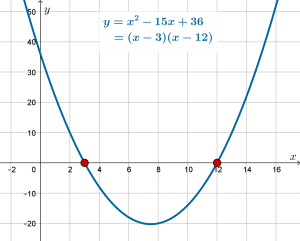The figure above is an example of two, real, distinct solutions to the equation.

A parabola may just touch the-axis, with the-axis being a tangent to the turning point of the graph: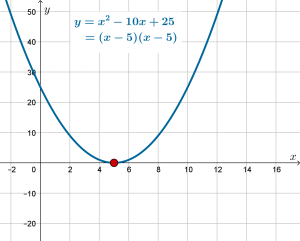The figure above is an example of there being one, real repeated solution to the equation.

A parabola may not cross the-axis at all:The figure above is an example of there being no real solutions to the equation.

In the context of graphing, solving a quadratic equation leads to the roots (-intercepts) of the parabola.

# Solving

When all three terms of the quadratic expression are present, we need to use factoring, the quadratic formula or the completing square method to solve.

We can solve linear equations more directly – we can generally isolate thejust by a sequence of adding, subtracting, multiplying, dividing both sides by the same quantity. However, in a quadratic equation we have both anterm and anterm which makes isolatingmore difficult.

# When there are only two terms, or if the expression factors

If a quadratic equation has only theterm and the constant, the equation is not difficult.

## Example (a)Also, if a quadratic equation  has only theterm and theterm, it can be factored.

## Example (b)The third kind of quadratic equation that is not difficult is the kind that factors easily.

## Example (c)In these three examples, there is no need for either the quadratic formula or the completed square method.

# Completing the Square

If there is no fast way to the solution then we must use either the quadratic formula or the completed square method.

## Example 1, expression onlyThe process is to introduce a square term (using half of, the coefficient of theterm) and then to subtract any new terms introduced by this process:We see that usinginstead ofwe have introduced an extra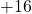. So we need to subtract thein order for our final line to equal our original line.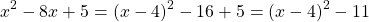Now let’s back up to make sure the right hand side is equal to the left hand side: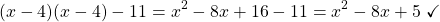ThereforeThis is called the completed square form. It is useful to us in the context of solving a quadratic equation because the unknownappears only once, which makes it possible to isolate.

# Using Completed Square to Solve

## Example 1

Now lets consider the equation. By completing the square we can solve as follows:## Example 2## Example 3# More Difficult: When the coefficient of theterm is not## Example 4

SolveBecause we have an equation, it is permitted to divide all terms byas follows: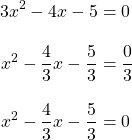We continue as follows:## Example 5

We can follow the same process if the coefficient ofis negative:## Example 6

SolveAs there are no real solutions to, we can conclude that there are no-intercepts to the parabola, and therefore, there are no real solutions to the equation.

# Practice

Practice using the completed square method to find the roots of these equations. Enter your answers correct to two decimal places.